# Determine surge and swab pressure method 2 Calculation Example

This section will show to how to use the formula from the previous topic, surge and swab pressure calculation 2nd method , to determine surge and swab pressure. I still use the same parameters as I used for the first method of surge and swab pressure calculation so you can compare the result between method#1 and method#2.

The well information is listed below:

θ300 = 85

θ600 = 130

Hole diameter = 9 inch

Drill Collar = 6.25 inch

ID of drill collar = 2.5 inch

Drillpipe diameter = 5 inch

ID of drill pipe = 3.5 inch

Average pipe running or pulling speed = 250 ft/min

Drill Pipe Length = 12000 ft

Drill Collar Length = 800 ft

Current Mud Weight = 12.5 ppg

Well TVD = 9000 ft

### Determine pressure loss around drill pipe

1. Determine fluid velocity around drill pipe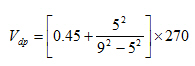Vdp = 224.1 ft/min

2. Maximum pipe velocity

Vm = 224.1 x 1.5 = 336 ft/min

3. Determine n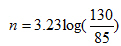n= 0.613

4. Determine K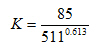K=1.863

5. Determine shear rate of drilling mud moving around drill pipe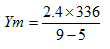Ym = 201.7

6. Determine shear stress of drilling mud moving around drill pipe

T= 1.863(201.7)0.613

T=48.1

7. Determine pressure loss around drill pipe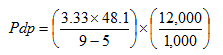Pdp = 480.5 psi.

### Determine pressure loss around drill collar

1. Determine fluid velocity around drill collar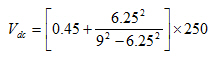Vdc = 345.4 ft/min

2. Maximum pipe velocity

Vm = 345.4 x 1.5 = 518 ft/min

3. Determine equivalent flow rate in gpm due to pipe movement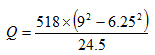Q=886.8 gpm

4. Determine pressure loss around drill collar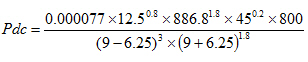Pdc = 71.8 psi

Note: PV = θ600 – θ300

### The summation of pressure loss around drill pipe and drill collar is total pressure loss.

Total pressure loss = 480.5 + 71.8 = 552.2 psi

## Surge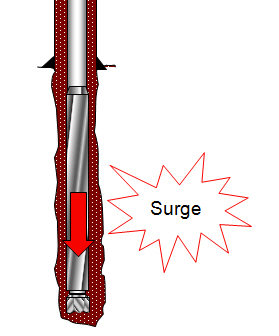Surge pressure = Hydrostatic pressure + Total pressure loss

Surge pressure = (0.052 x 9000 x 12.5) + 552.2 = 6402.2 psi

## Swab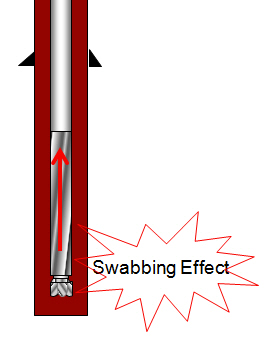Swab pressure = Hydrostatic pressure – Total pressure loss

Swab pressure = (0.052 x 9000 x 12.5) – 552.2 = 5297.8 psi

Since we use the same parameters, you compare the result to the first method, surge and swab pressure for close-ended pipe, surge pressure is 6476 psi and swab pressure is 5223 psi. As you can see, both methods figures within less than 2% different.

Share the joy